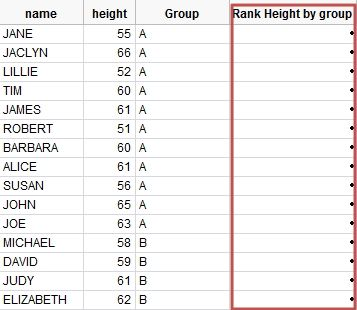Choose Language Hide Translation Bar
Highlighted

## JMP formula : Rank by group

JMP formula : Rank by group

Hi there~ ^^

Could you do me a favor?

-1. I want to use "Rank by Group"

-.I use JMP ver 9.02

I can use JMP function  "Col Rank(:height) "

but I cannot use this by groupping   "Col Rank(:height, :Group )"   Cannot typing this ":Group

-2. How can I ranking   up-to-down  or  down-to-up(default)  by height?1 ACCEPTED SOLUTION

Accepted Solutions
Highlighted

## Re: JMP formula : Rank by group

Hello,

As you have seen, there is currently no By option for Col Rank.  To accomplish the same thing, do the following:

1.  Add a column to store the original row order.  If you choose to use a formula with the Row() function, be sure to remove the formula after the values are stored in the column.

2.  Sort the table by height within Group.  You can choose to do this ascending or descending.

3.  Add a formula to the Rank Height by Group column that will assign a count which represents the rank within each group:

If(Row() == 1, 1, If(:Group[Row()] == :Group[Row() - 1], :Rank Height by Group[Row() - 1] + 1, 1))

4.  Sort the table by the original row order column.

Wendy Murphrey

Wendy
4 REPLIES 4
Highlighted

## Re: JMP formula : Rank by group

Hello,

As you have seen, there is currently no By option for Col Rank.  To accomplish the same thing, do the following:

1.  Add a column to store the original row order.  If you choose to use a formula with the Row() function, be sure to remove the formula after the values are stored in the column.

2.  Sort the table by height within Group.  You can choose to do this ascending or descending.

3.  Add a formula to the Rank Height by Group column that will assign a count which represents the rank within each group:

If(Row() == 1, 1, If(:Group[Row()] == :Group[Row() - 1], :Rank Height by Group[Row() - 1] + 1, 1))

4.  Sort the table by the original row order column.

Wendy Murphrey

Wendy
Highlighted

## Re: JMP formula : Rank by group

Wendy Murphrey,

I appreciate you taking the time to answer my questions!

and I'm happy to receive this answer from the author of 'Jump into JMP Scripting' book.

It was really helpful to understand JSL for begineer like me ^^

I hope you have a great day!

Highlighted

## Re: JMP formula : Rank by group

I was trying to solve this same problem and created a script that I could attach to my toolbar. Since there wasn't a single formula that can be easily applied, this script will manually assign the proper ranking to the current data table after it prompts the user for the relevant columns. Feel free to use or adapt if desired.

``````dt1 = Current Data Table();

nw = New Window( "Select Columns",
<<modal,
Text Box( "Select the Column you want to Rank by:" ),
selectRankBy = Col List Box(
all,
width( 250 ),
maxSelected( 1 )
),
Text Box( "Select the Column you want to Group by:" ),
selectGroupBy = Col List Box(
all,
width( 250 ),
maxSelected( 1 )
),
Text Box( "Input the Name of the new Column" ),
newNameBox = Text Edit Box( "New Column Name")
);
rankCol = Column( dt1, selectRankBy << Get Selected);
groupCol = Column( dt1, selectGroupBy << Get Selected);
newColName = newNameBox << Get Text;

allColumnNames = dt1 << Get Column Names( String );
If( Contains( allColumnNames, newColName ) == 0,
newCol = dt1 << New Column( newColName,
Numeric,
Continuous,
Format( "Best", 10 ),
<<Set Each Value( Empty() )
),
Column( newColName ) << Set Each Value( Empty() );
newCol = Column( newColName );
);
groupType = groupCol << Get Data Type;
uniqueGroups = Associative Array( groupCol << GetValues ) << GetKeys;

For( i = 1, i <= Length( uniqueGroups ), i++,
If( groupType == "Character",
Eval(
Parse(
"groupRows = dt1<< Get Rows Where(:" || (groupCol << Get Name())|| " ==\!"" || Char( uniqueGroups[i] ) || "\!")"
)
),
Eval(
Parse(
"groupRows = dt1<< Get Rows Where(:" || (groupCol << Get Name()) || " =="|| Char( uniqueGroups[i] ) || ")"
)
)
);
rowRankList = As List( Ranking( rankCol[groupRows] ) );
For( j = 1, j <= Length( rowRankList ), j++,
newCol[groupRows[j]] = rowRankList[j];
);
);``````
Highlighted

## Re: JMP formula : Rank by group

will this work for creaking the col rank by descending order too?

Article Labels

There are no labels assigned to this post.